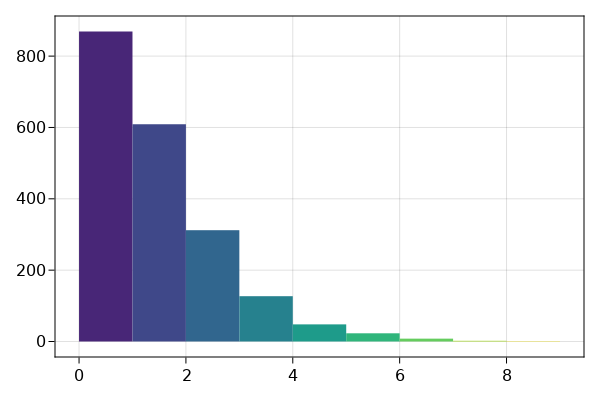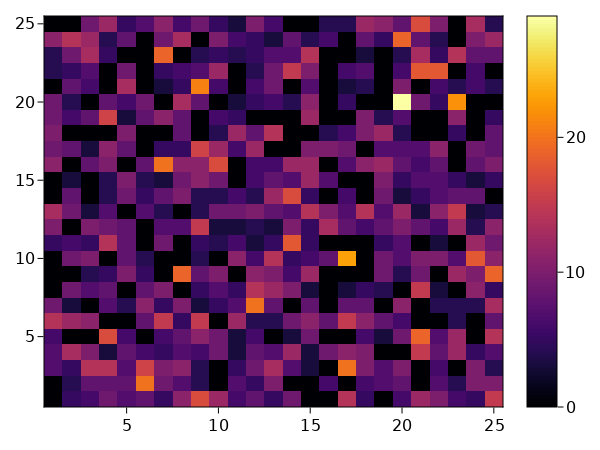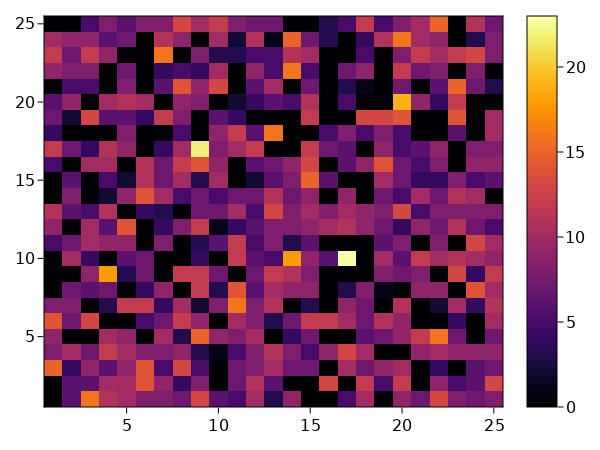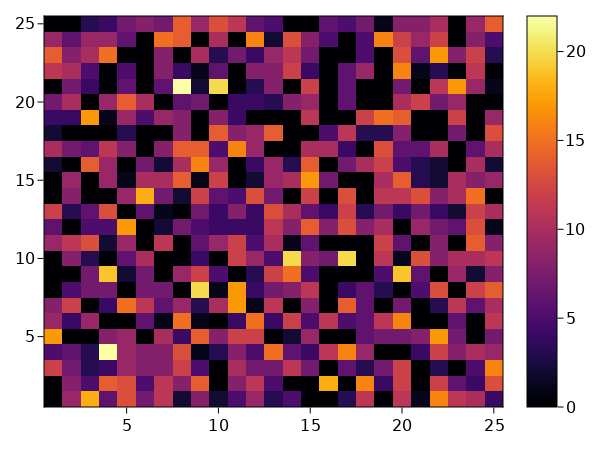# Wealth distribution model

This model is a simple agent-based economy that is modelled according to the work of Dragulescu et al.. This work introduces statistical mechanics concepts to study wealth distributions. What we show here is also referred to as "Boltzmann wealth distribution" model.

This model has a version with and without space. The rules of the space-less game are quite simple:

1. There is a pre-determined number of agents.
3. At every step an agent gives 1 unit of wealth (if they have it) to some other agent.

Even though this rule-set is simple, it can still recreate the basic properties of wealth distributions, e.g. power-laws distributions.

## Core structures: space-less

We start by defining the Agent type and initializing the model.

using Agents

mutable struct WealthAgent <: AbstractAgent
id::Int
wealth::Int
end

Notice that this agent does not have a pos field. That is okay, because there is no space structure to this example. We can also make a very simple AgentBasedModel for our model.

function wealth_model(; numagents = 100, initwealth = 1)
model = ABM(WealthAgent, scheduler = Schedulers.randomly)
for i in 1:numagents
end
return model
end

model = wealth_model()
AgentBasedModel with 100 agents of type WealthAgent
space: nothing (no spatial structure)
scheduler: randomly

The next step is to define the agent step function

function agent_step!(agent, model)
agent.wealth == 0 && return # do nothing
ragent = random_agent(model)
agent.wealth -= 1
ragent.wealth += 1
end

We use random_agent as a convenient way to just grab a second agent. (this may return the same agent as agent, but we don't care in the long run)

## Running the space-less model

Let's do some data collection, running a large model for a lot of time

N = 5
M = 2000
model = wealth_model(numagents = M)
data, _ = run!(model, agent_step!, N; adata)
data[(end-20):end, :]

21 rows × 3 columns

stepidwealth
Int64Int64Int64
1519801
2519810
3519820
4519831
5519840
6519850
7519861
8519873
9519881
10519891
11519900
12519910
13519923
14519930
15519940
16519951
17519960
18519972
19519982
20519990
21520000

What we mostly care about is the distribution of wealth, which we can obtain for example by doing the following query:

wealths = filter(x -> x.step == N - 1, data)[!, :wealth]
2000-element Vector{Int64}:
0
2
2
0
0
1
2
1
0
1
⋮
3
0
1
0
0
3
2
1
1

and then we can make a histogram of the result. With a simple visualization we immediately see the power-law distribution:

using CairoMakie
hist(
wealths;
bins = collect(0:9),
width = 1,
figure = (resolution = (600, 400),),
)## Core structures: with space

We now expand this model to (in this case) a 2D grid. The rules are the same but agents exchange wealth only with their neighbors.

It is also available from the Models module as Models.wealth_distribution.

We therefore have to add a pos field as the second field of the agents:

mutable struct WealthInSpace <: AbstractAgent
id::Int
pos::NTuple{2,Int}
wealth::Int
end

function wealth_model_2D(; dims = (25, 25), wealth = 1, M = 1000)
space = GridSpace(dims, periodic = true)
model = ABM(WealthInSpace, space; scheduler = Schedulers.randomly)
for i in 1:M # add agents in random positions
end
return model
end

model2D = wealth_model_2D()
AgentBasedModel with 1000 agents of type WealthInSpace
space: GridSpace with size (25, 25), metric=chebyshev, periodic=true
scheduler: randomly

The agent actions are a just a bit more complicated in this example. Now the agents can only give wealth to agents that exist on the same or neighboring positions (their "neighbors").

function agent_step_2d!(agent, model)
agent.wealth == 0 && return # do nothing
neighboring_positions = collect(nearby_positions(agent.pos, model))
push!(neighboring_positions, agent.pos) # also consider current position
rpos = rand(model.rng, neighboring_positions) # the position that we will exchange with
available_ids = ids_in_position(rpos, model)
if length(available_ids) > 0
random_neighbor_agent = model[rand(model.rng, available_ids)]
agent.wealth -= 1
random_neighbor_agent.wealth += 1
end
end

## Running the model with space

init_wealth = 4
model = wealth_model_2D(; wealth = init_wealth)
data, _ = run!(model, agent_step_2d!, 10; adata = adata, when = [1, 5, 9])
data[(end-20):end, :]

21 rows × 4 columns

stepidwealthpos
Int64Int64Int64Tuple…
199802(20, 5)
299811(3, 4)
399821(13, 22)
499830(20, 11)
599844(2, 6)
699856(3, 8)
799860(15, 23)
899874(11, 6)
999885(19, 3)
10998920(10, 21)
1199902(21, 4)
1299910(14, 11)
1399920(11, 24)
1499933(13, 1)
1599946(8, 2)
16999510(5, 2)
1799966(20, 19)
1899977(23, 10)
1999986(15, 11)
20999911(20, 1)
21910000(13, 14)

Okay, now we want to get the 2D spatial wealth distribution of the model. That is actually straightforward:

function wealth_distr(data, model, n)
W = zeros(Int, size(model.space))
for row in eachrow(filter(r -> r.step == n, data)) # iterate over rows at a specific step
W[row.pos...] += row.wealth
end
return W
end

function make_heatmap(W)
figure = Figure(; resolution = (600, 450))
hmap_l = figure[1, 1] = Axis(figure)
hmap = heatmap!(hmap_l, W; colormap = cgrad(:default))
cbar = figure[1, 2] = Colorbar(figure, hmap; width = 30)
return figure
end

W1 = wealth_distr(data, model2D, 1)
make_heatmap(W1)W5 = wealth_distr(data, model2D, 5)
make_heatmap(W5)W10 = wealth_distr(data, model2D, 9)
make_heatmap(W10)What we see is that wealth gets more and more localized.